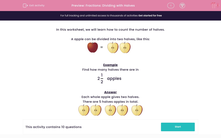# Fractions: Dividing with Halves

In this worksheet, students evaluate the number of halves. Cakes are used to illustrate this concept.Key stage:  KS 2

Curriculum topic:   Maths and Numerical Reasoning

Curriculum subtopic:   Fractions

Difficulty level:#### Worksheet Overview

In this worksheet, we will learn how to count the number of halves.

A apple can be divided into two halves, like this:=Example

Find how many halves there are in

2

 1 2

apples

Each whole apple gives two halves.

There are 5 halves apples in total.### What is EdPlace?

We're your National Curriculum aligned online education content provider helping each child succeed in English, maths and science from year 1 to GCSE. With an EdPlace account you’ll be able to track and measure progress, helping each child achieve their best. We build confidence and attainment by personalising each child’s learning at a level that suits them.

Get started